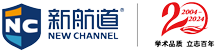4008-125-888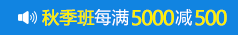# sat数学真题及答案解析（5）

2020-05-12 11:23来源：互联网作者：上海管理员

1. Which of the following could be a value of x, in the diagram above?

A. 10

B. 20

C. 40

D. 50

E. any of the above

The marked angle, ABC must be more than 90 degrees because it is the external angle of triangle BDC, and must be equal to the sum of angles BDC (90) and DCB. Also ABC is not a straight line and must be less than 180. Therefore 90 < 5x < 180 The only value of x which satisfies this relation is 20.

2. Helpers are needed to prepare for the fete. Each helper can make either 2 large cakes or 35 small cakes per hour. The kitchen is available for 3 hours and 20 large cakes and 700 small cakes are needed. How many helpers are required?

A. 10

B. 15

C. 20

D. 25

E. 30

20 large cakes will require the equivalent of 10 helpers working for one hour. 700 small cakes will require the equivalent of 20 helpers working for one hour. This means if only one hour were available we would need 30 helpers. But since three hours are available we can use 10 helpers.

3. Jo's collection contains US, Indian and British stamps. If the ratio of US to Indian stamps is 5 to 2 and the ratio of Indian to British stamps is 5 to 1, what is the ratio of US to British stamps?

A. 5 : 1

B. 10 : 5

C. 15 : 2

D. 20 : 2

E. 25 : 2

Indian stamps are common to both ratios. Multiply both ratios by factors such that the Indian stamps are represented by the same number. US : Indian = 5 : 2, and Indian : British = 5 : 1. Multiply the first by 5, and the second by 2.

Now US : Indian = 25 : 10, and Indian : British = 10 : 2 Hence the two ratios can be combined and US : British = 25 : 2

4. A 3 by 4 rectangle is inscribed in circle. What is the circumference of the circle?

A. 2.5π

B. 3π

C. 5π

D. 4π

E. 10π

Draw the diagram. The diagonal of the rectangle is the diameter of the circle. The diagonal is the hypotenuse of a 3,4,5 triangle, and is therefore, 5. Circumference = π.diameter = 5π

5. Two sets of 4 consecutive positive integers have exactly one integer in common. The sum of the integers in the set with greater numbers is how much greater than the sum of the integers in the other set?

A. 4

B. 7

C. 8

D. 12

E. it cannot be determined from the information given.

If two sets of four consecutive integers have one integer in common, the total in the combined set is 7., and we can write the sets as n + (n + 1) + (n + 2) + (n + 3 ) and (n + 3) + (n + 4) + (n + 5) + (n + 6) Note that each term in the second set is 3 more than the equivalent term in the first set. Since there are four terms the total of the differences will be 4 x 3 = 12

# 免费领取资料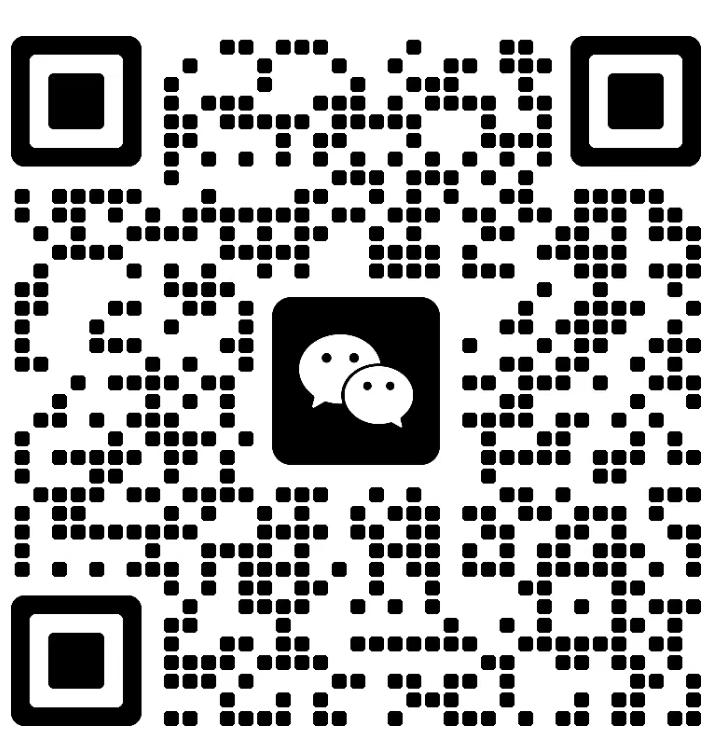### 热门课程

• SAT考前模考冲刺班
• 走读班（3-6人）
• 住宿班（3-6人）
• SAT一对一

SAT考前模考冲刺班 40课时 ￥3800 在线咨询
SAT十一特训班 6-8人 48课时 ￥16800 在线咨询
SAT模考刷题班 不限 4天 ￥5800 在线咨询

SAT强化班（3-6人，走读） 3-6人 64课时 ￥21800 在线咨询
SAT冲刺班（3-6人.走读） 3-6人 32课时 ￥11800 在线咨询
SAT预备班（3-6人，走读） 3-6人 32课时 ￥10800 在线咨询
SAT基础班（3-6人，走读） 3-6人 64课时 ￥20800 在线咨询

SAT一对一 1 按需定制 在线咨询

#### 相关文章推荐

1、如转载本网原创文章，请表明出处;
2、本网转载媒体稿件旨在传播更多有益信息，并不代表同意该观点，本网不承担稿件侵权行为的连带责任；
3、如本网转载稿、资料分享涉及版权等问题，请作者见稿后速与新航道联系（电话：021-64380066），我们会第一时间删除。

#### SAT30天热搜

• 徐家汇校区
• 人民广场校区
• 浦东校区
• 中山公园校区
• 杨浦校区
• 闵行校区
• 松江校区
• 封闭集训营
• 地址：徐汇区裕德路126号

乘车路线：地铁1、4号线上海体育馆、3号线9号线宜山路站、11号线上海游泳馆站

• 地址：南京西路338号天安中心24楼

乘车路线：地铁1、2、8号线人民广场站

• 地址：浦东新区世纪大道1128号岚桥国际大厦3楼（地铁2号线世纪大道站12号口出）

乘车路线：地铁2、4、6号线世纪大道站

• 地址：长宁路1158号 贝多芬广场 A座4楼414室

乘车路线：乘车路线：地铁3、4号线中山公园站

• 地址：杨浦区国宾路18号万达广场A座18楼

乘车路线：地铁10号线五角场站

• 地址：东川路1779-19号

乘车路线：地铁5号线 东川路站4号口出

• 地址：松江大学城四期校区：文汇路928弄想飞天地2204

乘车路线：地铁9号线松江大学城站下

• 地址：上海市浦东新区惠南镇拱极路2151号

乘车路线：地铁16号线惠南站

•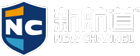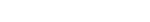•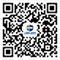微信公众号
•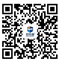微信社群

+86

+86

### 收不到验证码？

• 1.请检查是否输入正确的手机号码
• 2.检查手机是否停机
• 3.请使用其他账号登录
• 4.请联系官方客服

+86